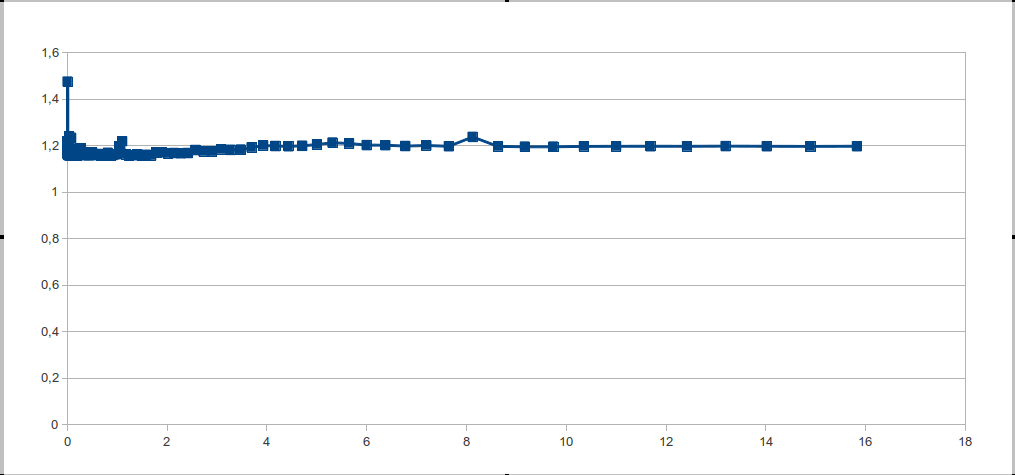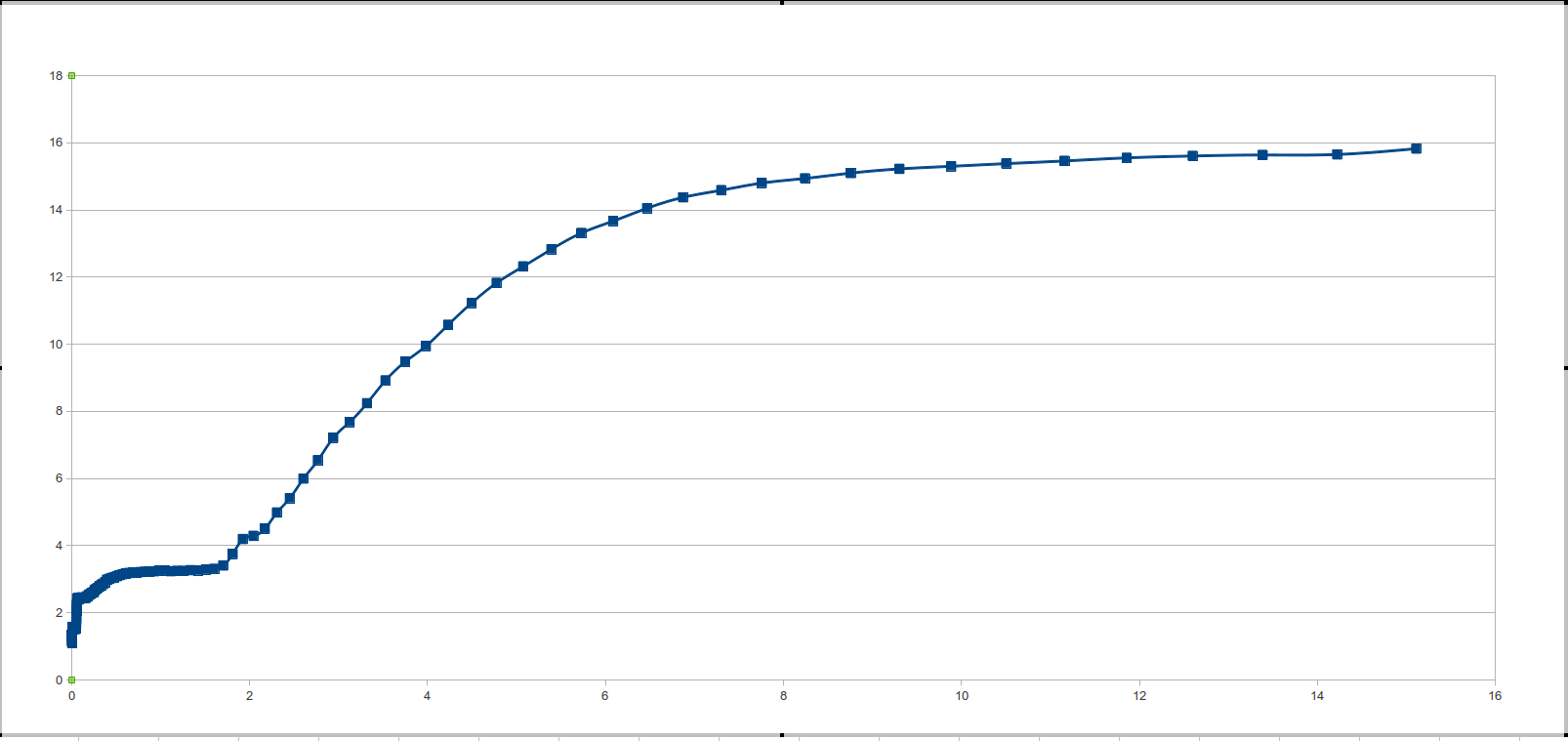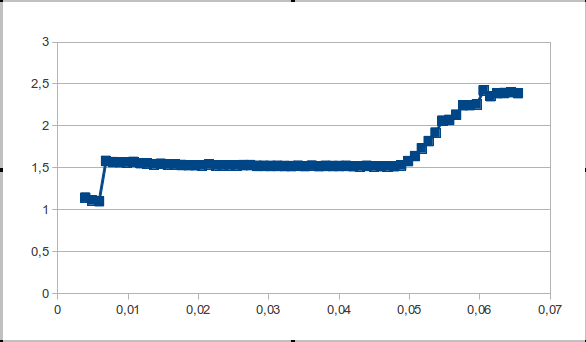Experimentation of cache characteristics

Due to what, then, are we seeing a constant increase in the productivity of single-threaded programs? At the moment, we are at that stage in the development of microprocessor technologies, when the increase in the speed of single-threaded applications depends only on memory. The number of cores is growing, but the frequency was fixed within 4 GHz and does not give a performance gain.

The speed and frequency of memory operation is the main thing due to which we get “our own piece of a free cake” (link). That is why it is important to use memory as efficiently as we can, and even more so as fast as the cache. To optimize the program for a specific computer, it is useful to know the characteristics of the processor cache: the number of levels, size, line length. This is especially important in high-performance code - system kernels, mathematical libraries.

How to determine the characteristics of the cache automatically? (Naturally, cpuinfo is not considered to be parsed, at least because in the end we would like to get an algorithm that can be easily implemented in other OSs. It’s convenient, isn't it?) That’s what we’ll do now.

Bit of theory

There are currently and widely used three types of cache: direct mapping cache, associative cache, and multi-associative cache.

Direct mapping cache
- this line of RAM can be displayed in a single line of the cache, but many possible lines of RAM can be displayed in each line of the cache.Associative cache
- any line of RAM can be mapped to any line of the cache.Multiple Associative Cache
- the cache memory is divided into several “banks”, each of which functions as a cache with direct mapping, so the RAM line can be displayed not in the only possible cache entry (as it would be in the case of direct mapping), but in one of several banks ; the bank is selected on the basis of LRU or another mechanism for each line placed in the cache.LRU - crowding out the “longest unused” line, memory cache.

Idea

To determine the number of cache levels, you need to consider the order of memory access, on which the transition will be clearly visible. Different cache levels differ primarily in memory response speed. In the case of a “cache miss” for the L1 cache, data will be searched in the following memory levels, and if the data size is larger than L1 and less than L2, then the memory response speed will be the L2 response speed. The previous statement is also true in general cases.

It is clear that you need to choose a test on which, we will clearly see the miss cache and test it on various data sizes.

Knowing the logic of multiple-associative caches working according to the LRU algorithm, it is not difficult to come up with an algorithm on which the cache "falls", nothing tricky is to pass along a line. The efficiency criterion is the time of one memory access. Naturally, you need to consistently apply to all elements of the string, repeating repeatedly to average the result. For example, there may be cases when the line fits in the cache but for the first pass we load the line from RAM and therefore we get completely inadequate time.

I would like to see something like stairs, passing through rows of different lengths. To determine the nature of the steps, we consider an example of line-passing for the direct and associative cache, the case with a multiple-associative cache will be the average between the direct-mapping cache and the associative cache.

Associative Cache
As soon as the size of the data exceeds the size of the cache memory, the
fully associative cache “misses” each time the memory is accessed.Direct cache
Consider different line sizes. - Shows the maximum number of misses that the processor will spend to access the elements of the array the next pass on the line.As you can see, the memory access time does not increase sharply, but as the amount of data increases. As soon as the size of the data exceeds the size of the cache, there will be misses with every access to the memory.

Therefore, in the associative cache, the step will be vertical, and in the direct cache, it will gradually increase up to a double size of the cache. Multiple associative caches will be the middle case, the “cusp,” if only because access time cannot be better than direct.

If we talk about memory, then the fastest is the cache, followed by the operational one, the slowest is swap, we will not talk about it in the future. In turn, different cache levels (as a rule today processors have 2-3 cache levels) have different memory response speeds: the higher the level, the lower the response speed. And therefore, if a line is placed in the first level of the cache, (which by the way is completely associative), the response time will be less than that of a line significantly exceeding the size of the first-level cache. Therefore, on the graph of the response time of the memory from the size of the line there will be several plateaus - a plateau * of the memory response, and a plateau caused by different levels of the cache.

*Плато функции — { i:x, f(xi) — f(xi+1) < eps: eps → 0 }

Let's get started

For implementation we will use C (ANSI C99).

Quickly written code, the usual passage through the lines of different lengths, less than 10mb, performed repeatedly. (We will give small pieces of the program that carry a semantic load).

for (i = 0; i < 16; i++) {
for (j = 0; j < L_STR; j++) A[j]++;
}

We look at the chart and we see one big step. But in theory everything turns out, just wonderful. So you need to understand: what is this happening for? And how to fix it?Obviously, this can happen for two reasons: either the processor does not have a memory cache, or the processor guesses memory accesses so well. Since the first option is closer to fiction, the reason for everything is a good prediction of hits.

The fact is that today far from top-end processors, in addition to the principle of spatial locality, also predict arithmetic progression in the order of access to memory. Therefore, you need to randomize memory accesses.

The length of the randomized array must be comparable with the length of the main line in order to get rid of the large granularity of calls, the length of the array should not be a power of two, because of this there were “overlays” - which may result in outliers. It is best to set the granularity constant, including if the granularity is a prime, then the effects of overlays can be avoided. And the length of a randomized array is a function of the length of the string.

for (i = 0; i < j; i++) {
for (m = 0; m < L; m++) {
for (x = 0; x < M; x++){
v = A[ random[x] + m ];
}
}
}

After that, we surprised such a long-awaited "picture", which was discussed at the beginning.The program is divided into 2 parts - test and data processing. Write a script in 3 lines to run or run 2 times with pens yourself.

Listing file size. With Linux

#include	<stdio.h>
#include	<stdlib.h>
#include	<string.h>
#include	<time.h>

#define		T	char
#define		MAX_S	0x1000000
#define		L	101

volatile T A[MAX_S];
int m_rand[0xFFFFFF];

int main (){
static struct timespec t1, t2;

memset ((void*)A, 0, sizeof (A));

srand(time(NULL));

int v, M;
register int i, j, k, m, x;

for (k = 1024; k < MAX_S;) {
M = k / L;

printf("%g\t", (k+M*4)/(1024.*1024));

for (i = 0; i < M; i++) m_rand[i] = L * i;
for (i = 0; i < M/4; i++)	{
j = rand() % M;
x = rand() % M;

m = m_rand[j];
m_rand[j] = m_rand[i];
m_rand[i] = m;

}

if (k < 100*1024) j = 1024;
else if (k < 300*1024) j = 128;
else j = 32;

clock_gettime (CLOCK_PROCESS_CPUTIME_ID, &t1);
for (i = 0; i < j; i++) {

for (m = 0; m < L; m++) {
for (x = 0; x < M; x++){
v = A[ m_rand[x] + m ];
}
}

}
clock_gettime (CLOCK_PROCESS_CPUTIME_ID, &t2);

printf ("%g\n",1000000000. * (((t2.tv_sec + t2.tv_nsec * 1.e-9) - (t1.tv_sec + t1.tv_nsec * 1.e-9)))/(double)(L*M*j));

if (k > 100*1024)	k += k/16;
else			k += 4*1024;
}
return 0;
}

Listing file size. With Windows

#include	<stdio.h>
#include	<stdlib.h>
#include	<string.h>
#include	<time.h>
#include	<iostream>
#include	<Windows.h>
using namespace std;

#define		T	char
#define		MAX_S	0x1000000
#define		L	101

volatile T A[MAX_S];
int m_rand[0xFFFFFF];

int main (){
LARGE_INTEGER freq; LARGE_INTEGER time1; 	LARGE_INTEGER time2;
QueryPerformanceFrequency(&freq);

memset ((void*)A, 0, sizeof (A));

srand(time(NULL));

int v, M;
register int i, j, k, m, x;

for (k = 1024; k < MAX_S;) {
M = k / L;

printf("%g\t", (k+M*4)/(1024.*1024));

for (i = 0; i < M; i++) m_rand[i] = L * i;
for (i = 0; i < M/4; i++)	{
j = rand() % M;
x = rand() % M;

m = m_rand[j];
m_rand[j] = m_rand[i];
m_rand[i] = m;

}

if (k < 100*1024) j = 1024;
else if (k < 300*1024) j = 128;
else j = 32;

QueryPerformanceCounter(&time1);
for (i = 0; i < j; i++) {

for (m = 0; m < L; m++) {
for (x = 0; x < M; x++){
v = A[ m_rand[x] + m ];
}
}

}
QueryPerformanceCounter(&time2);

printf ("%g\n",1000000000. * span/(double)(L*M*j));

if (k > 100*1024)	k += k/16;
else			k += 4*1024;
}
return 0;
}

In general, I think everything is clear, but I would like to stipulate a few points.

Array A is declared as volatile - this directive guarantees us that there will always be calls to array A, that is, they will not be "cut" by either the optimizer or the compiler. It’s also worth mentioning that the entire computational load is performed before measuring time, which allows us to reduce the background effect.

The file is translated into assembler on Ubuntu 12.04 and the gcc 4.6 compiler - the cycles are saved.

Data processing

For data processing it is logical to use derivatives. And despite the fact that with an increase in the order of differentiation, the noise increases, the second derivative and its properties will be used. No matter how noisy the second derivative is, we are only interested in the sign of the second derivative.

We find all the points at which the second derivative is greater than zero (with some error because the second derivative, in addition to being considered numerically, is very noisy). We define the function of the dependence of the sign of the second derivative of the function on the cache size. The function takes a value of 1 at points where the sign of the second derivative is greater than zero, and zero if the sign of the second derivative is less than or equal to zero.

The “take-off” points are the beginning of each rung. Also, before processing the data, it is necessary to remove single outliers that do not change the semantic load of the data, but create noticeable noise.

Listing file data_pr.s

#include <stdio.h>
#include <stdlib.h>
#include <math.h>

double round (double x)
{
int mul = 100;
if (x > 0)
return floor(x * mul + .5) / mul;
else
return ceil(x * mul - .5) / mul;
}

float size, time, der_1, der_2;

int main(){
size = 0; time = 0; der_1 = 0; der_2 = 0;
int i, z = 110;
for (i = 1; i < 110; i++)
scanf("%g%g", &size[i], &time[i]);

for (i = 1; i < z; i++)
der_1[i] = (time[i]-time[i-1])/(size[i]-size[i-1]);

for (i = 1; i < z; i++)
if ((time[i]-time[i-1])/(size[i]-size[i-1]) > 2)
der_2[i] = 1;
else
der_2[i] = 0;

//comb
for (i = 0; i < z; i++)
if (der_2[i] == der_2[i+2] && der_2[i+1] != der_2[i]) der_2[i+1] = der_2[i];

for (i = 0; i < z-4; i++)
if (der_2[i] == der_2[i+3] && der_2[i] != der_2[i+1] && der_2[i] != der_2[i+2]) {
der_2[i+1] = der_2[i]; der_2[i+2] = der_2[i];
}

for (i = 0; i < z-4; i++)
if (der_2[i] == der_2[i+4] && der_2[i] != der_2[i+1] && der_2[i] != der_2[i+2] && der_2[i] != der_2[i+3]) {
der_2[i+1] = der_2[i]; der_2[i+2] = der_2[i]; der_2[i+3] = der_2[i];
}
//

int k = 1;

for (i = 0; i < z-4; i++){
if (der_2[i] == 1) printf("L%d = %g\tMb\n", k++, size[i]);
while (der_2[i] == 1) i++;
}

return 0;
}

Tests

CPU / OS / kernel version / compiler / compilation keys - will be indicated for each test.

• Intel Pentium CPU P6100 @ 2.00GHz / Ubuntu 12.04 / 3.2.0-27-generic / gcc -Wall -O3 size.c -lrt
L1 = 0.05 Mb
L2 = 0.2 Mb
L3 = 2.7 Mb
• I will not give all the good tests, let's talk about the Rake better

A rake was detected during data processing on the Intel Xeon 2.4 / L2 = 512 kB / Windows Server 2008 server processor.
The problem is a small number of points falling on the plateau exit interval - accordingly, the jump of the second derivative is invisible and is taken as noise.

You can solve this problem by the least squares method, or run tests in the process of determining plateau zones.

I would like to hear your suggestions regarding a solution to this problem.

List of references

• Makarov A.V. Computer Architecture and Low-Level Programming.
• Ulrich Drepper What every programmer should know about memory